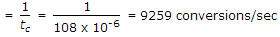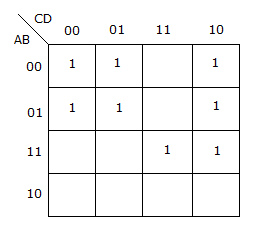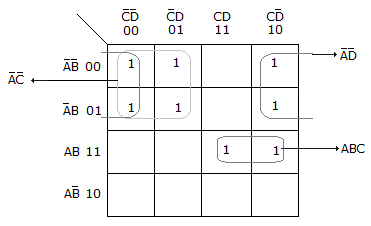# Electronics and Communication Engineering - Digital Electronics

26.

The Boolean expression A ⊕ B is equivalent to

 A. AB + AB B. AB + AB C. B D. A

Explanation:

A ⊕ B = AB + AB

27.

Which of these are two state devices?

 A. Lamp B. Punched card C. Magnetic tape D. All of the above

Explanation:

Each has 2 states.

28.

What will be minimum conversion rate in 6 bit dual slope A/D converter uses a reference of -6v and a 1 MHz clock. It uses a fixed count of 40 (101000).

 A. 9000 B. 9259 C. 1000 D. 1000

Explanation:

Minimum conversion rate.

29.

The minimum number of NAND gates required to implement the Boolean function A +AB + ABC is equal to

 A. 0 B. 1 C. 4 D. 7

Explanation:

A + AB +A B C = A + AB ( 1 + C) = A + AB = A(1 + B) = A.

30.

For the K map of the given figure, the simplified Boolean expression isA. A C + A D + ABC B. A B D + BC C. A C D + AC D. A C D + AC + BC